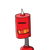# if tanvi gives an interest of Rs.40 for one year at 5% rate p.a. What is the sum she has borrowed​

if tanvi gives an interest of Rs.40 for one year at 5% rate p.a. What is the sum she has borrowed​

### 1 thought on “if tanvi gives an interest of Rs.40 for one year at 5% rate p.a. What is the sum she has borrowed​”

1.## Solution:-

━━━━━━━━━━━━━━━━━━━━━━━━━━

$$\large\to{\underbrace{\underline{\sf{Understanding\:the\:concept:-}}}}$$

◍ Here, the question has given us the simple interest, rate of interest and time that is Rs. 40, 5% per annum and for 1 year respectively. Now, the question has asked us to find the sum she has borrowed it means we have to find the principal. So to get the answer we simply have to apply the formula of SI by taking Principal as x and we will easily get our answer.

◈ The principal is Rs. 800.

### GIVEN:-

☆ Simple Interest = Rs. 40

☆ Rate of Interest = 5% per annum

☆ Time = 1 year

### TO FIND:-

↠Principal = ?

### FORMULA:-

➾ Simple Interest = P × R × T / 100 [For finding the principal.]

### SOLVING BY APPLYING THE FORMULA:-

☆ Simple Interest = Rs. 40

☆ Rate of Interest = 5% per annum

☆ Time = 1 year

➾ Simple Interest = P × R × T / 100

• Finding the principal:
• Let the principal be x.

➢ 40 = x × 5 × 1 / 100

➢ 40 = x × 5 / 100

➢ Taking 100 to L.H.S.

➢ 40 × 100 = x × 5

➢ 4000 = x × 5

➢ 4000 = 5x

➢ 4000 / 5 = x

➢ 800 = x

➢ x = 800

Hence, we got the answer. The principal is of Rs. 800.

━━━━━━━━━━━━━━━━━━━━━━━━━━